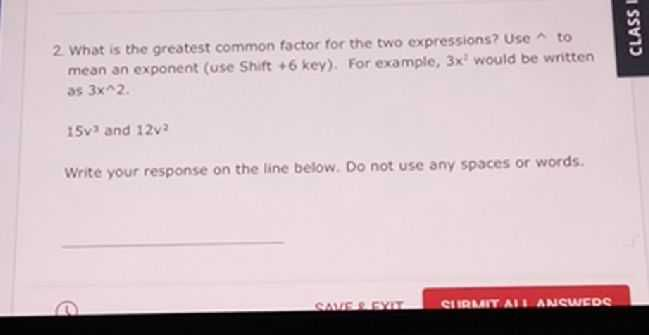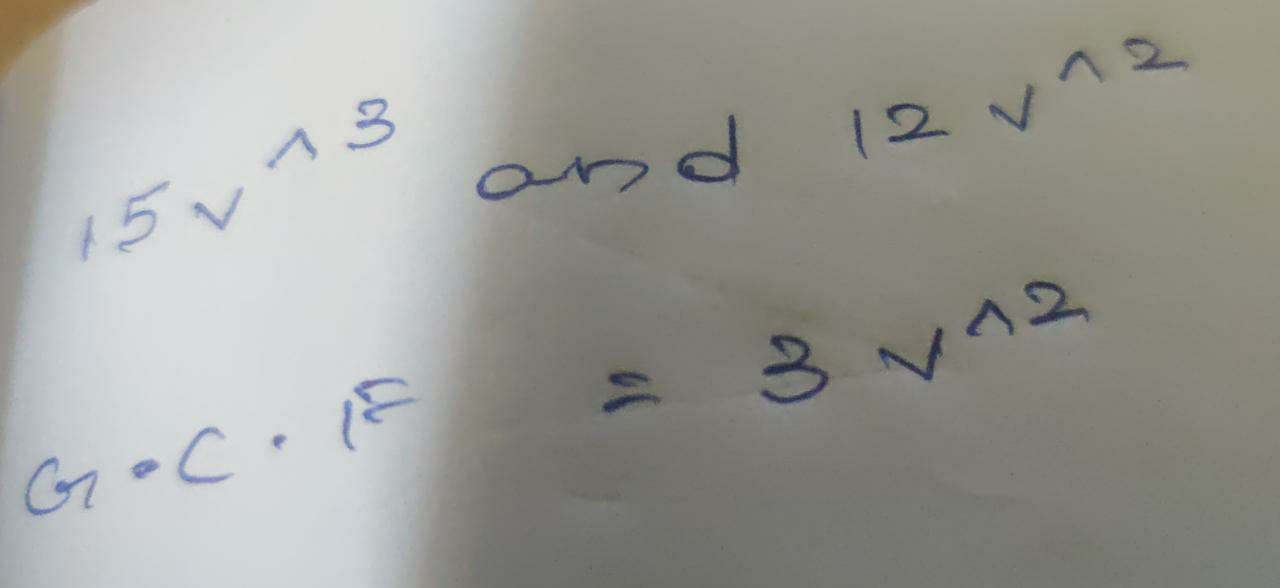### Still have math questions?

Algebra
QuestionWhat is the greatest common factor for the two expressions? Use A to mean an exponent (use Shift + $$6$$ key). For example, $$3 \times ^ { 2 }$$ would be written as $$3 \times n _ { 2 }$$ .

$$15 v ^ { 3 }$$ and $$12 v ^ { 2 }$$

Write your response on the line below. Do not use any spaces or words.